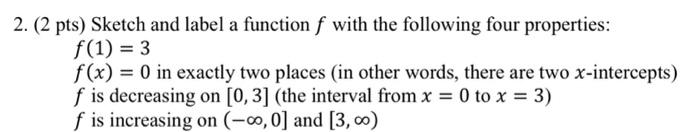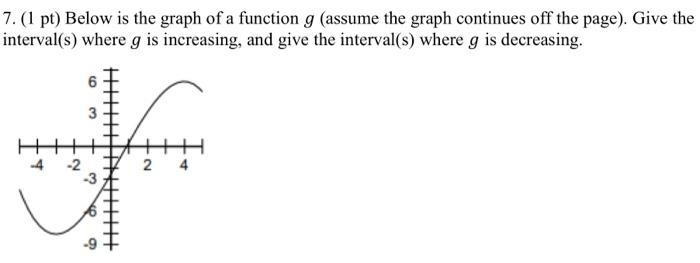Home / Expert Answers / Calculus / 2-2-pts-sketch-and-label-a-function-f-with-the-following-four-properties-f-1-3-pa222

# (Solved): 2. ( 2 pts) Sketch and label a function $$f$$ with the following four properties: $f(1)=3$ $$...2. ( 2 pts) Sketch and label a function \( f$$ with the following four properties: $f(1)=3$ $$f(x)=0$$ in exactly two places (in other words, there are two $$x$$-intercepts) $$f$$ is decreasing on $$[0,3]$$ (the interval from $$x=0$$ to $$x=3$$ ) $$f$$ is increasing on $$(-\infty, 0]$$ and $$[3, \infty)$$ 7. (1 pt) Below is the graph of a function $$g$$ (assume the graph continues off the page). Give the interval(s) where $$g$$ is increasing, and give the interval(s) where $$g$$ is decreasing.

We have an Answer from Expert